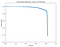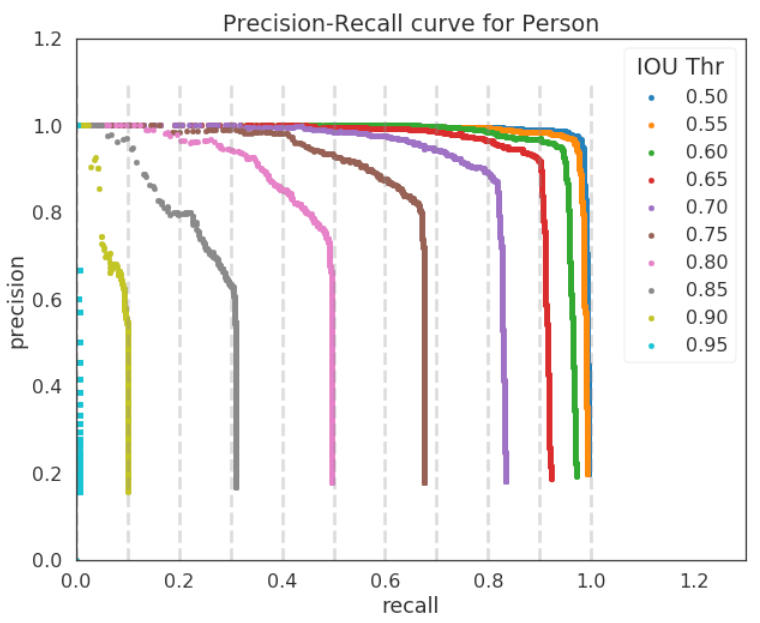# Understanding the mAP Evaluation Metric for Object Detection

If you’ve evaluated models in object detection or you’ve read papers in this area, you may have encountered the mean average precision or “mAP score” (for example here or here or here). It has become the accepted way to evaluate object detection competitions, such as for the PASCAL VOC, ImageNet, and COCO challenges. In this article, I will explain:

• what the mean average precision (mAP) metric is,
• why it is a useful metric in object detection,
• how to calculate it with example data for a particular class of object.

Additionally, I will provide some code (link at the end of the article) to compute this metric for use in your own projects or work if desired.

In object detection, evaluation is non trivial, because there are two distinct tasks to measure:

1. Determining whether an object exists in the image (classification)
2. Determining the location of the object (localization, a regression task).

Furthermore, in a typical data set there will be many classes and their distribution is non-uniform (for example there might be many more dogs than ice cream cones). So a simple accuracy-based metric will introduce biases. It is also important to assess the risk of misclassifications. Thus, there is the need to associate a “confidence score” or model score with each bounding box detected and to assess the model at various level of confidence.

In order to address these needs, the Average Precision (AP) was introduced. To understand the AP, it is necessary to understand the precision and recall of a classifier. For a more comprehensive explanation of these terms, the wikipedia article is a nice place to start. Briefly, in this context, precision measures the “false positive rate” or the ratio of true object detections to the total number of objects that the classifier predicted. If you have a precision score of close to 1.0 then there is a high likelihood that whatever the classifier predicts as a positive detection is in fact a correct prediction. Recall measures the “false negative rate” or the ratio of true object detections to the total number of objects in the data set. If you have a recall score close to 1.0 then almost all objects that are in your dataset will be positively detected by the model. Finally, it is very important to note that the there is an inverse relationship between precision and recall and that these metrics are dependent on the model score threshold that you set (as well as of course, the quality of the model). For example, in this image from the TensorFlow Object Detection API, if we set the model score threshold at 50 % for the “kite” object, we get 7 positive class detections, but if we set our model score threshold at 90 %, there are 4 positive class detections.

To calculate the AP, for a specific class (say a “person”) the precision-recall curve is computed from the model’s detection output, by varying the model score threshold that determines what is counted as a model-predicted positive detection of the class. An example precision-recall curve may look something like this for a given classifier:Precision-Recall curve for an example classifier. A point on the precision-recall curve is determined by considering all objects above a given model score threshold as a positive prediction, then calculating the resulting precision and recall for that threshold.

The final step to calculating the AP score is to take the average value of the precision across all recall values (see explanation in section 4.2 of the Pascal Challenge paper pdf which I outline here). This becomes the single value summarizing the shape of the precision-recall curve. To do this unambiguously, the AP score is defined as the mean precision at the set of 11 equally spaced recall values, Recall_i = [0, 0.1, 0.2, …, 1.0]. Thus,

The precision at recall i is taken to be the maximum precision measured at a recall exceeding Recall_i.

Up until now, we have been discussing only the classification task. For the localization component (was the object’s location correctly predicted?) we must consider the amount of overlap between the part of the image segmented as true by the model vs. that part of the image where the object is actually located.

In order to evaluate the model on the task of object localization, we must first determine how well the model predicted the location of the object. Usually, this is done by drawing a bounding box around the object of interest, but in some cases it is an N-sided polygon or even pixel by pixel segmentation. For all of these cases, the localization task is typically evaluated on the Intersection over Union threshold (IoU). For definiteness, throughout the rest of the article, I’ll assume that the model predicts bounding boxes, but almost everything said will also apply to pixel-wise segmentation or N-sided polygons. Many good explanations of IoU exist, (see this one for example), but the basic idea is that it summarizes how well the ground truth object overlaps the object boundary predicted by the model.

Model object detections are determined to be true or false depending upon the IoU threshold. This IoU threshold(s) for each competition vary, but in the COCO challenge, for example, 10 different IoU thresholds are considered, from 0.5 to 0.95 in steps of 0.05. For a specific object (say, ‘person’) this is what the precision-recall curves may look like when calculated at the different IoU thresholds of the COCO challenge:Precision-Recall curves calculated at various IoU thresholds, according to the COCO challenge. Dashed lines correspond to equally spaced recall values where the AP is calculated.

Now that we’ve defined Average Precision (AP) and seen how the IoU threshold affects it, the mean Average Precision or mAP score is calculated by taking the mean AP over all classes and/or over all IoU thresholds, depending on the competition. For example:

• PASCAL VOC2007 challenge only 1 IoU threshold was considered: 0.5 so the mAP was averaged over all 20 object classes.
• For the COCO 2017 challenge, the mAP was averaged over all 80 object categories and all 10 IoU thresholds.

Averaging over the 10 IoU thresholds rather than only considering one generous threshold of IoU ≥ 0.5 tends to reward models that are better at precise localization.

# Code for Calculating the mean Average Precision

I found the code for calculating the mean Average Precision in the COCO dataset a bit opaque and perhaps not well-optimized. So I created my own set of functions to perform the calculation without relying on the coco API(for bounding boxes only at this time). The code takes ground truth boxes in the format of a dictionary of lists of boxes:

`{"filename1": [[xmin, ymin, xmax, ymax],...,[xmin, ymin, xmax, ymax]],"filename2": [...],...}`

and predicted boxes as a dictionary of a dictionary of boxes and scores like this:

`{'filename1': {  'boxes': [[xmin, ymin, xmax, ymax],...,[xmin, ymin, xmax, ymax]],  'scores': [score1,...,scoreN]}, 'filename2': {   'boxes': [[xmin, ymin, xmax, ymax],...,[xmin, ymin, xmax, ymax]],   'scores': [score1,...,scoreN]},...}`

For the example I was working with, I had a total of 656 ground truth boxes to evaluate for one category (person) and a total number of 4854 predicted boxes for the same category (person), and it takes me a total of ~0.45 seconds to calculate the AP at 1 IoU threshold for 1 class (running on my laptop with 16 GB or RAM and a 3.1 GHz Intel Core processor). See the code on github for details, and thanks for reading!

Machine Learning Lead at Just Auto Insurance. Former Astrophysicist.

## More from Timothy C Arlen

Machine Learning Lead at Just Auto Insurance. Former Astrophysicist.